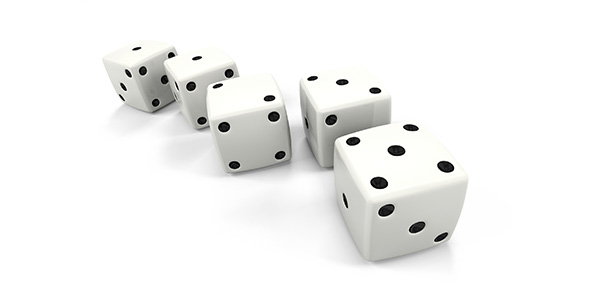# Probability And Stats Quiz (12.1-12.6)

10 Questions | Attempts: 114
ShareSettingsThis quiz covers the fundamental counting principle, permutations, combinations, probability, and statistical measures.

• 1.
State whether the following events are mutually exclusive or inclusive: Selecting a face card or a diamond
• A.

Mutually exclusive

• B.

Inclusive

• 2.
How many distinct arrangements are there of the letters in the word CALCULUS?
• 3.
In how many ways can I arrange 10 books on a shelf if the shelf only holds 7 books and the dictionary must be the first book?
• 4.
There are 28 students in your Algebra 2 class. In how many ways can we choose a 4 person math competition team?
• 5.
Two dice are rolled. What is the probability that the sum of the dice is a multiple of 3 (round to the nearest hundredth)?
• 6.
A coin is flipped four times. What is the probability of getting 2 heads and 2 tails (round to three decimals)?
• 7.
Mrs. Pek has a box full of different colored compasses. There are 8 green, 10 pink, 5 yellow, and 11 orange compasses. What is the probability that she chooses a green compass followed by an orange, followed by another green compass if she does not replace the first two compasses? Round to the nearest three decimal places.
• 8.
You are creating a password for your computer login. The password must be 1 letter followed by 2 digits followed by 2 letters. How many passwords are possible if the letters cannot be repeated and the digits 0 or 9 cannot be used?
• 9.
Set up the equation for
• A.
• B.
• C.
• D.
• 10.
Find the standard deviation (to the nearest tenth) of the following set of data: {2.4, 5.6, 1.9, 7.1, 4.3, 2.7, 4.6, 1.8, 2.4}

## Related TopicsBack to top
×

Wait!
Here's an interesting quiz for you.